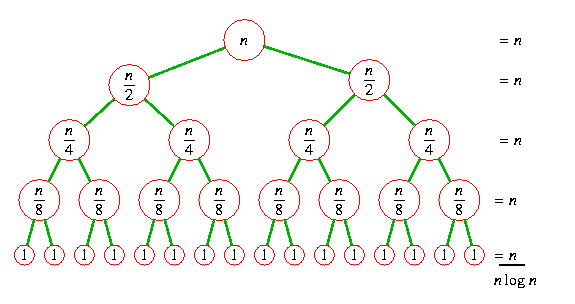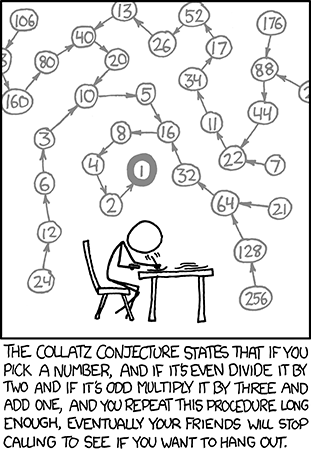Sign-up for a presentation slot

## Daily Challenge

Note the "fast version" and the "slow version"

Thinking Task 1: Calculate the 26th fibonacci number. How much larger was it than the 25th? Without using code, which fibonacci number do you think will be the first to be larger than 1 billion?

Thinking Task 2: The first method starts to break down around 34-35. Count out the length of time it takes to calculate the 34th fib. Estimate how long it would take to calculate the 44th fib.

Comparison Task 3: Why does the 2nd method take so many less additions? How long does it take to calculate the 44th fib with the 2nd method? When do you think it will start to break?

# Class 11: Recursion 2

So last time we dealt with a "base case" for when `n == 0` or `n == cutoff`. Check out this type of "base case":

Interpret Task 4: What is the "base case"? What is the "recursive step"?

Here is a generic "structural recursion" template for the list case and the int case:

List Sum Task 5: Write a recursive function to add all of the values in a list.

Reverse String Task 6: Follow this pattern to write a function which consumes a string a produces the string backwards.

## Generative Recursion

Sometimes the steps aren't as obvious as `n-1` or `input[1:]`.

How Task 7: How did this code work? Explain it to your neighbor.

## Divide and ConquerSo if you can make your problem half-sized then you can make things very fast!

Here is a sorted list of random integers:

Is in Task 8: How many items do you need to look at to decide if 123456 is in that list?

Here is a powerful recursive idea called binary search:

Observe Task 9: How many searches did the divide and conquer approach need to find various elements? How much smaller is the problem each size? How big of a problem do you think this approach can tackle?Put it together task 10: Make the tests pass in the following code snippet. `"".join(L)` concatenates all the elements in the list `L`.

## Daily ChallengeDaily Collatz Challenge: Implement a recursive algorithm which consumes an integer `n` and if `n == 1` returns the number of iterations if it is even calls itself on `n/2` if it is odd calls itself on ```3n + 1```. Find the longest collatz sequence starting at an `n` less than 1000.

Extra Credit Solve https://projecteuler.net/problem=14